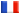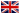# Séminaire de Cryptographie

## Damien Robert### Computing isogenies of small degrees on Abelian Varieties

Isogenies are an essential tool in Elliptic Curves cryptography, where they are used in a wide variety of area: fast point counting, complex multiplication methods... Velu's formulas give an efficient method for computing such isogenies, but there are no formula known for curves of higher genera or general abelian varieties (except some special case for isogenies of degree 2). In this talk we will present the framework of the theta structure on an abelian variety, developped by Mumford in 1967, which allows us to compute isogenies. For this we lift a theta null point of level $l$, corresponding to an abelian variety B, to the modular space of theta null points of level $lk$. We use a specialized Groebner algorithm that considerably speed-up this phase, and we show how to detect degenerate solutions. To each lifted point corresponds an isogeny of degree $\pi: A \to B$ of degree $k$. We then explain how to compute their dual efficiently.

This is a joint work with Jean-Charles Faugere and David Lubicz.